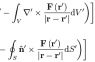# Triple integral with a curl

• I

In the image attached to this post, there is an equation on the top line and one on the bottom line. In the proof this image was taken from, they say this is a consequence of divergence theorem but I'm not quite understanding how it is. If anyone could explicitly explain the process to go from the top equation to the bottom, that would be greatly appreciated!

#### Attachments

•Screen Shot 2016-04-17 at 8.58.03 PM.png
2 KB · Views: 547

Consider a vector field
$$\mathbf{G} = \mathbf{c \times F}$$
where $$\mathbf{c}$$ is an arbitrary constant vector. Apply the divergence theorem to $$\mathbf{G}$$ and I think you can reconstruct the result.

jason

Consider a vector field
$$\mathbf{G} = \mathbf{c \times F}$$
where $$\mathbf{c}$$ is an arbitrary constant vector. Apply the divergence theorem to $$\mathbf{G}$$ and I think you can reconstruct the result.

jason

To clarify, would ##\mathbf{G}## replace the entire integrand in the top line of my image (including the curl) since that itself is a vector? I can see the general line of reasoning now, but how is it deduced that ##\nabla ' \times \mathbf {\frac{F(r')}{\big|{r-r'}\big|} } = \mathbf{G} = \mathbf{\hat{n}}' \times \mathbf {\frac{F(r')}{\big|{r-r'}\big|} }##? The curl of this vector should definitely be normal, to itself and I think I'm missing something fundamental here, but why is it necessarily normal to the surface, too?

Last edited:
I don't think my post was clear enough, so let me start over. Please ignore my previous post.

I am attempting to show you how to derive the general expression, for which you can insert you special case. Define a vector field $\mathbf{P(r) = c \times Q(r) }$. Now write down the Divergence theorem
$$\int \int \mathbf{P(r^\prime) \cdot \hat{n}^\prime} dS^\prime = \int \int \int \mathbf{\nabla^\prime \cdot P(r^\prime) dV^\prime }$$
You now need to simplify this. You should use the fact that $\mathbf{c}$ is a constant so that $\mathbf{\nabla \times c=0}$
in order to simplify the expression. Then use standard vector algebra to arrange to get the $\mathbf{c}$ outside of both integrals. In the end you should find,
$$\mathbf{c \cdot} \left[ \int \int \mathbf{Q(r^\prime) \times \hat{n}^\prime} dS^\prime + \int \int \int \mathbf{\nabla^\prime \times Q(r^\prime) }dV^\prime \right] = 0.$$
Since $\mathbf{c}$ is arbitrary we must have,
$$\int \int \mathbf{\hat{n}^\prime \times Q(r^\prime)} dS^\prime = \int \int \int \mathbf{\nabla^\prime \times Q(r^\prime) }dV^\prime.$$
Does that make sense?

Jason

I don't think my post was clear enough, so let me start over. Please ignore my previous post.

I am attempting to show you how to derive the general expression, for which you can insert you special case. Define a vector field $\mathbf{P(r) = c \times Q(r) }$. Now write down the Divergence theorem
$$\int \int \mathbf{P(r^\prime) \cdot \hat{n}^\prime} dS^\prime = \int \int \int \mathbf{\nabla^\prime \cdot P(r^\prime) dV^\prime }$$
You now need to simplify this. You should use the fact that $\mathbf{c}$ is a constant so that $\mathbf{\nabla \times c=0}$
in order to simplify the expression. Then use standard vector algebra to arrange to get the $\mathbf{c}$ outside of both integrals. In the end you should find,
$$\mathbf{c \cdot} \left[ \int \int \mathbf{Q(r^\prime) \times \hat{n}^\prime} dS^\prime + \int \int \int \mathbf{\nabla^\prime \times Q(r^\prime) }dV^\prime \right] = 0.$$
Since $\mathbf{c}$ is arbitrary we must have,
$$\int \int \mathbf{\hat{n}^\prime \times Q(r^\prime)} dS^\prime = \int \int \int \mathbf{\nabla^\prime \times Q(r^\prime) }dV^\prime.$$
Does that make sense?

Jason

Thank you! That makes sense. :)